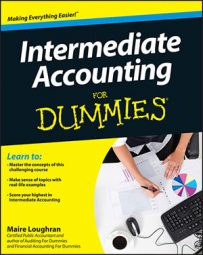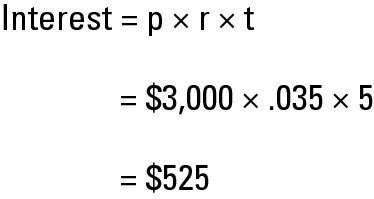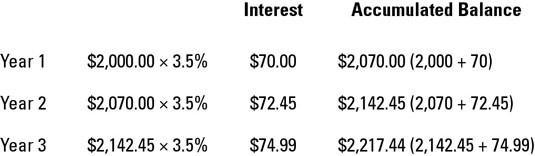##### Intermediate Accounting For DummiesWhat’s the difference between simple and compound interest, anyway? It’s important to have at least a basic understanding of how a company or bank determines the interest rate you earn on your money on deposit.

Basically, the two major criteria to setting interest rates are the riskiness of the investment and what rate is commonly being paid. For example, if you have a good credit score, you’ll receive a more favorable interest rate when borrowing money to make a purchase than someone who has horrible credit.

Or if your bank needs to beef up its money on deposit, it may pay a higher interest rate than the competition, to attract new customers.

## How to calculate simple interest

You figure simple interest on the principal, which is the amount of money borrowed or on deposit using a basic formula: Principal x Rate x Time (Interest = p x r x t). Your intermediate accounting textbook may substitute n for time — the n stands for number of periods (time).

Say your brother wants to buy a used car for \$5,000 and has only \$2,000 for the down payment. He hits you up for a loan for the remaining \$3,000. If the length of the loan is five months and he’s paying you simple interest of 3.5 percent per month to borrow the additional \$3,000, your interest income equals \$525.Simple interest is used only for loans and investments of less than one year. If the time is longer than one year, compound interest applies instead.

## How to calculate compound interest

Hold on to your hats! Now that you understand the basic calculation for simple interest, it’s time to familiarize yourself with how to figure compound interest, which really shows the time value of money. You figure compound interest on both the amount of principal and any interest earned but not withdrawn.

For example, let’s say that your brother decides not to replace his old car and instead invests the \$2,000 proposed down payment, earning 3.5 percent interest. Using the theory of compound interest, he earns interest each month on the amount of principal and interest the bank pays him for his money on deposit — in other words, the accumulated balance.

Any lending institution that’s required to abide by federal law, such as a bank, must state its interest rates annually and as compound rather than simple interest.As you can see, the calculations are a bit more involved than when figuring simple interest. Luckily, banks and other financial institutions that perform these calculations regularly have software for the job.

Your intermediate accounting textbook provides five interest tables to help you compute the time value of money. Two tables deal with a single sum; three address annuities, which is a series of payments.

If you don’t want to have to crack open your huge intermediate accounting textbook every time you want to check out these interest tables, you’ll be glad to know that you can also find them online. Do a search using the key phrase “present and future value tables” to find a plethora of options. You can also use a financial calculator or an Excel function on your computer.

Your intermediate accounting textbook also shows the formulas the tables are built on. You can just use those formulas, if you want, although the tables are much easier to work with.